# Order By / Sorting Results in SQL

ORDER BY clause is used to sort the records in the table either in ascending or descending order. We can even use this clause on the complex query results. Sorting can be done on one or more columns of the table or query result. When columns are sorted by listing them in ORDER BY clause, those columns should also be present in SELECT list.

Consider the simple SELECT query on BOOKS table.

`SELECT * FROM BOOKS;`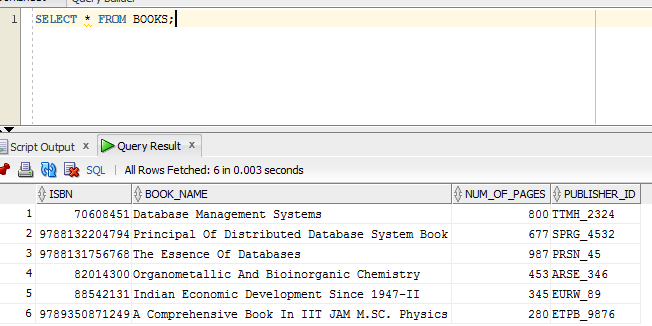What we can see here is simple SELECT query results in the records in the order they are inserted. It does not sort the records according any columns or primary key. If we need to see the result in sorted order (either ascending or descending) of any particular column, then we need to explicitly specify the columns in the ORDER BY clause. Suppose we need to sort the results based on book number – ISBN, then we need to write query as shown below.

`SELECT * FROM BOOKS ORDER BY ISBN;`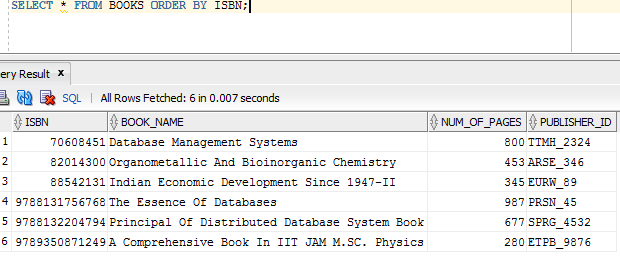It displays the result in sorted order of ISBN. It by default sorts the results in ascending order. If we need to sort the results based on some other column, say BOOK_NAME, then we can specify it in the same way as below.

`SELECT * FROM BOOKS ORDER BY BOOK_NAME;`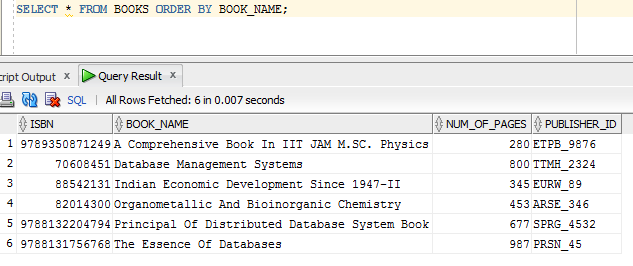This query also sorts the results in a ascending order of BOOK_NAME. If we need to sort the results in descending order then we need to specify a keyword DESC at the end of the column listed in the ORDER BY clause like below.

`SELECT * FROM BOOKS ORDER BY BOOK_NAME DESC;`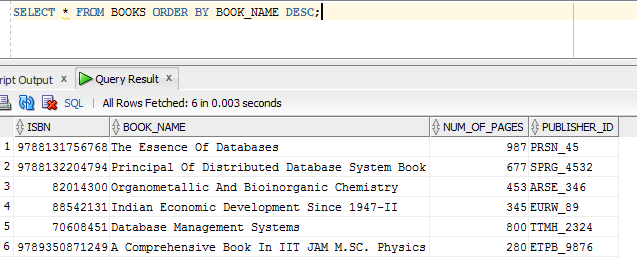We can have any number of columns listed in the ORDER BY clause to sort them, provided they are also present in SELECT list. We can individually specify whether we want to sort each of the columns in ascending or descending order. By default, all the columns in ORDER BY clause are sorted in ascending order. If we need any specific column to be sorted in descending order, then we have to add DESC after that column. Suppose we need to sort the result of BOOKS table by ascending order of BOOK_NAME and descending order of ISBN, then the query is as shown below:

`SELECT * FROM BOOKS ORDER BY BOOK_NAME, ISBN DESC;`

Or

`SELECT * FROM BOOKS ORDER BY ISBN DESC, BOOK_NAME;`

Are these queries result in same result? No. Here order of the columns listed in ORDER BY clause also important. It sorts the results in the order they are listed in the ORDER BY clause. That means in the first query above, it sorts the BOOK_NAME in ascending order first, and then it sorts its ISBN in descending order. Whereas in the second query, it sorts all the ISBNs in descending order first, then sorts their names in ascending order. Hence we can see different results for both of them. Hence order of the columns in the sorting is also important.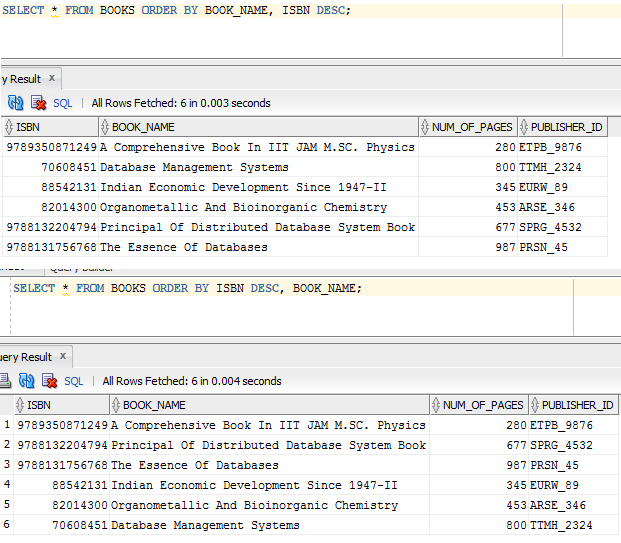ORDER BY clause need not be used in simple queries, but can also be used in any complex queries too. Consider the below query to display all the ISBN, BOOK_NAME, copy of the book, and its loan / due dates which has been borrowed by the borrower. It shows the result in the ascending order of ISBN and its copy number.

```SELECT b.ISBN, b.BOOK_NAME, bl.BOOK_CP_NUMBER, bl.LOAN_DATE, bl.DUE_DATE
FROM BOOKS b, BOOK_LOAN bl, BOOK_COPY bc
WHERE b.ISBN = bl.ISBN
AND b.ISBN = bc.ISBN
AND bl.BOOK_CP_NUMBER = bc.COPY_NUM
ORDER BY b.ISBN, bl.BOOK_CP_NUMBER;```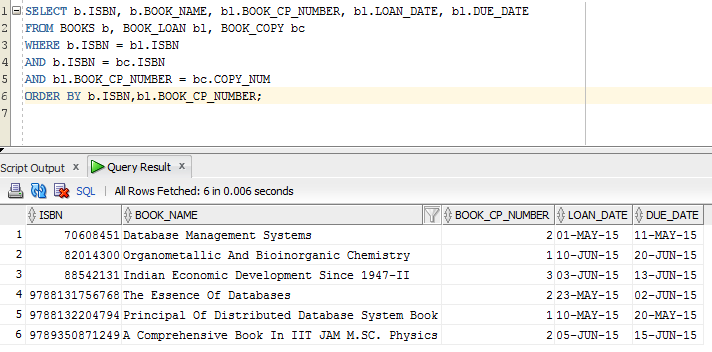### Below query is same as above but it displays the result in descending order of ISBN, but in ascending order of its copy.

```SELECT b.ISBN, b.BOOK_NAME, bl.BOOK_CP_NUMBER, bl.LOAN_DATE, bl.DUE_DATE
FROM BOOKS b, BOOK_LOAN bl, BOOK_COPY bc
WHERE b.ISBN = bl.ISBN
AND b.ISBN = bc.ISBN
AND bl.BOOK_CP_NUMBER = bc.COPY_NUM
ORDER BY b.ISBN DESC, bl.BOOK_CP_NUMBER;```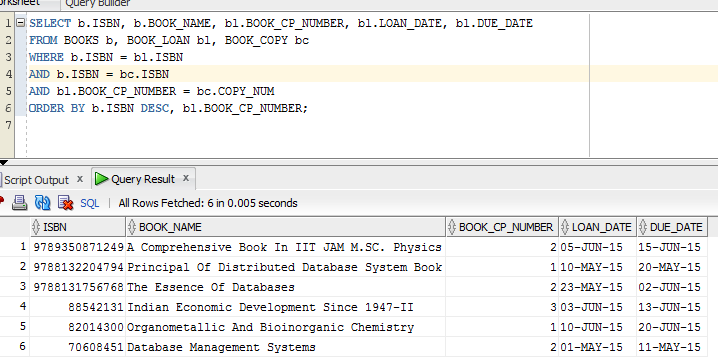Below query shows the same query as above, but order of columns in sorting lists are changed, which resulted in different order in the result.

```SELECT b.ISBN, b.BOOK_NAME, bl.BOOK_CP_NUMBER, bl.LOAN_DATE, bl.DUE_DATE
FROM BOOKS b, BOOK_LOAN bl, BOOK_COPY bc
WHERE b.ISBN = bl.ISBN
AND b.ISBN = bc.ISBN
AND bl.BOOK_CP_NUMBER = bc.COPY_NUM
ORDER BY, bl.BOOK_CP_NUMBER, b.ISBN DESC;```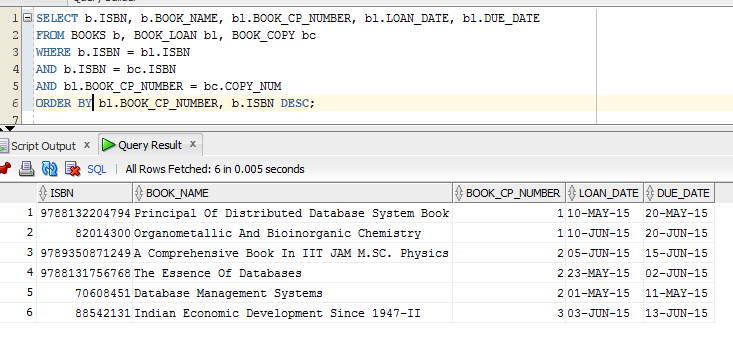In above all cases, we have seen that sorting is done based on ascending or descending order of alphabets or numbers. We can even use ORDER BY clause on dates and times columns. In addition, if we need to sort the results in our own defined way, then we can sort it too.

Consider the query to display the books based on the category. But order of the category is user defined as shown below. It sorts category name in different order that user has specified.

```SELECT c.CATEGORY_NAME, b.BOOK_NAME
FROM CATEGORY c, BOOK_CATEGORY bc, BOOKS b
WHERE c.CATEGORY_ID = bc.CATEGORY_ID
AND bc.ISBN = b.ISBN
ORDER BY (CASE c.CATEGORY_NAME WHEN 'Database' THEN 1
WHEN 'Physics' THEN 2
WHEN 'Chemistry' THEN 3
WHEN 'Economics' THEN 4
ELSE 50 END), b.BOOK_NAME;```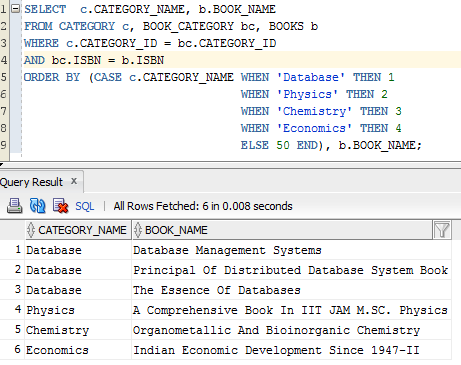We can specify them to sort in descending order too.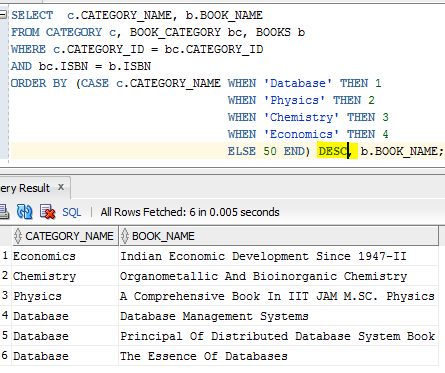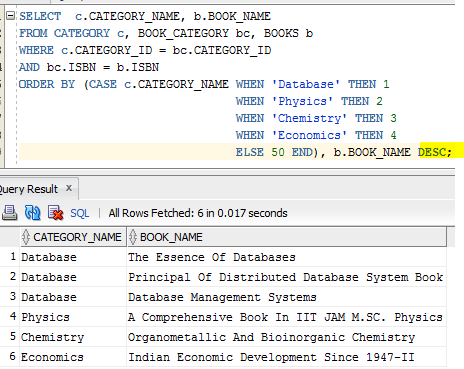Translate »